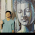VHDL coding tips and tricks: VHDL code for a simple ALU

## Thursday, June 23, 2011

### VHDL code for a simple ALU

ALU(Arithmetic Logic Unit) is a digital circuit which does arithmetic and logical operations. Its a basic block in any processor.
In this article I have shared vhdl code for a simple ALU. Note that this is one of the simplest architecture of an ALU. Most of the ALU's used in practical designs are far more complicated and requires good design experience.
The block diagram of the ALU is given below. As you can see, it receives two input operands 'A' and 'B' which are 8 bits long. The result is denoted by 'R' which is also 8 bit long. The input signal 'Op' is a 3 bit value which tells the ALU what operation to be performed by the ALU. Since 'Op' is 3 bits long we can have 2^3=8 operations.

Our ALU is capable of doing the following operations:

ALU OperationDescription
Add SignedR = A + B : Treating A, B, and R as signed two's complement integers.
Subtract SignedR = A - B : Treating A, B, and R as signed  two's complement integers.
Bitwise ANDR(i) = A(i) AND B(i).
Bitwise NORR(i) = A(i) NOR B(i).
Bitwise ORR(i) = A(i) OR B(i).
Bitwise NANDR(i) = A(i) NAND B(i).
Bitwise XORR(i) = A(i) XOR B(i).
Biwise NOTR(i) = NOT A(i).

These functions are implemented using a case statement. The ALU calculates the outputs at every positive edge of clock cycle. As soon as the outputs are calculated it is available at the port signal 'R'. See the code below, to know how it is done:

library IEEE;
use IEEE.STD_LOGIC_1164.ALL;
use IEEE.NUMERIC_STD.ALL;

entity simple_alu is
port(   Clk : in std_logic; --clock signal
A,B : in signed(7 downto 0); --input operands
Op : in unsigned(2 downto 0); --Operation to be performed
R : out signed(7 downto 0)  --output of ALU
);
end simple_alu;

architecture Behavioral of simple_alu is

--temporary signal declaration.
signal Reg1,Reg2,Reg3 : signed(7 downto 0) := (others => '0');

begin

Reg1 <= A;
Reg2 <= B;
R <= Reg3;

process(Clk)
begin

if(rising_edge(Clk)) then --Do the calculation at the positive edge of clock cycle.
case Op is
when "000" =>
Reg3 <= Reg1 + Reg2;  --addition
when "001" =>
Reg3 <= Reg1 - Reg2; --subtraction
when "010" =>
Reg3 <= not Reg1;  --NOT gate
when "011" =>
Reg3 <= Reg1 nand Reg2; --NAND gate
when "100" =>
Reg3 <= Reg1 nor Reg2; --NOR gate
when "101" =>
Reg3 <= Reg1 and Reg2;  --AND gate
when "110" =>
Reg3 <= Reg1 or Reg2;  --OR gate
when "111" =>
Reg3 <= Reg1 xor Reg2; --XOR gate
when others =>
NULL;
end case;
end if;

end process;

end Behavioral;

The testbench code used for testing the ALU code is given below:

LIBRARY ieee;
USE ieee.std_logic_1164.ALL;
USE ieee.numeric_std.ALL;

ENTITY tb IS
END tb;

ARCHITECTURE behavior OF tb IS

signal Clk : std_logic := '0';
signal A,B,R : signed(7 downto 0) := (others => '0');
signal Op : unsigned(2 downto 0) := (others => '0');
constant Clk_period : time := 10 ns;

BEGIN

-- Instantiate the Unit Under Test (UUT)
uut: entity work.simple_alu PORT MAP (
Clk => Clk,
A => A,
B => B,
Op => Op,
R => R
);

-- Clock process definitions
Clk_process :process
begin
Clk <= '0';
wait for Clk_period/2;
Clk <= '1';
wait for Clk_period/2;
end process;

-- Stimulus process
stim_proc: process
begin
wait for Clk_period*1;
A <= "00010010"; --18 in decimal
B <= "00001010"; --10 in decimal
Op <= "000";  wait for Clk_period; --add A and B
Op <= "001";  wait for Clk_period; --subtract B from A.
Op <= "010";  wait for Clk_period; --Bitwise NOT of A
Op <= "011";  wait for Clk_period; --Bitwise NAND of A and B
Op <= "100";  wait for Clk_period; --Bitwise NOR of A and B
Op <= "101";  wait for Clk_period; --Bitwise AND of A and B
Op <= "110";  wait for Clk_period; --Bitwise OR of A and B
Op <= "111";  wait for Clk_period; --Bitwise XOR of A and B
wait;
end process;

END;
This is the simulation waveform:

The code is sythesisable. I haven't used std_logic_vector in this code. You can read this post to know why.

The block diagram was adopted from this original article. To make sure you have understood the concepts well, try implementing the block diagram given in the original article.

#### 8 comments:

1.Thanks for posting such a nice code but please make it correct, i think simulation will give result only for op 111, to correct result in simulation you must remove wait statements from last part of test bench.

look like this

-- Stimulus process
stim_proc: process
begin

A <= "00010010"; --18 in decimal
B <= "00001010"; --10 in decimal
Op <= "000"; wait for Clk_period; --add A and B
Op <= "001"; wait for Clk_period; --subtract B from A.
po Op <= "010"; wait for Clk_period; --Bitwise NOT of A
Op <= "011"; wait for Clk_period; --Bitwise NAND of A and B
Op <= "100"; wait for Clk_period; --Bitwise NOR of A and B
Op <= "101"; wait for Clk_period; --Bitwise AND of A and B
Op <= "110"; wait for Clk_period; --Bitwise OR of A and B
Op <= "111"; wait for Clk_period; --Bitwise XOR of A and B

end process;

END;

2.why do we need a clk fo the alu isn't it a combinational block?

3.This comment has been removed by the author.

4.can we use A B C as it is instead of temp variables?

5.check out the Verilog version of this code here.
http://verilogcodes.blogspot.com/2015/10/verilog-code-for-simple-alu.html

6.it gives me error for "Wait For statement unsupported"
for all statements wherever wait for Clk_period; is written!!

7.I have a basys 3 I was wondering how I would write the top_ALU code for this?

8.if i want 1111100 shift in left about 1 what can i do ??